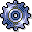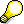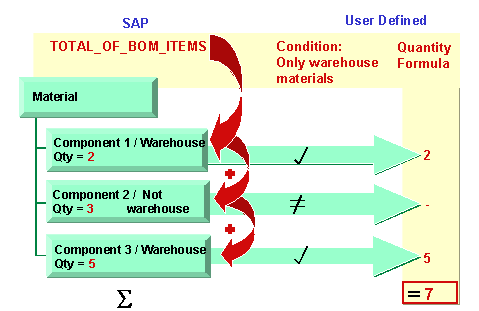Flexible FunctionsUse

Flexible functions significantly increase the options for quantity calculations by making it possible to run evaluations using internal tables. For example, internal tables can contain business transactions for routings, components of a BOM or costing items. The data accessed becomes more detailed. It is possible, for example, to evaluate several BOM components of a production order, or material components of a network activity.

Flexible functions also allow you to continue processing the results after checking specific, user-defined requirements.

Flexible functions consist of the following:

• Table that is evaluated (specified in a flexible function)
• Conditions: for each internal table entry, the R/3 System checks whether a user condition is met.
• Formula: if the condition is fulfilled, the R/3 System evaluates a user-defined formula (per entry in the internal table). The formula uses the data just located for the entry in question. The formula result is a numerical value.
• Calculation: all formula results of an evaluation run are then processed. They can, for example, be totalled, or the average can be calculated (specified in the flexible function). The description of the flexible function shows the type of processing: Total... - Addition, Multi ... - Multiplication, Average ... Average calculation.If you do not define a condition, the formula evaluates all entries from the internal table.

Activities

You define the flexible functions in the editor of the template. Choose a function with table assignment from the function tree, and enter the conditions and formulas.

For more information, see Defining Flexible Functions.

Example

A flexible function evaluates a BOM (TotalOfBOMItems) The system checks whether each BOM component fills the condition "item category = stock material" (condition is entered by the user). If the condition is filled, the values are calculated per component through a formula entered by the user. The individual values are calculated (specified in the flexible function).